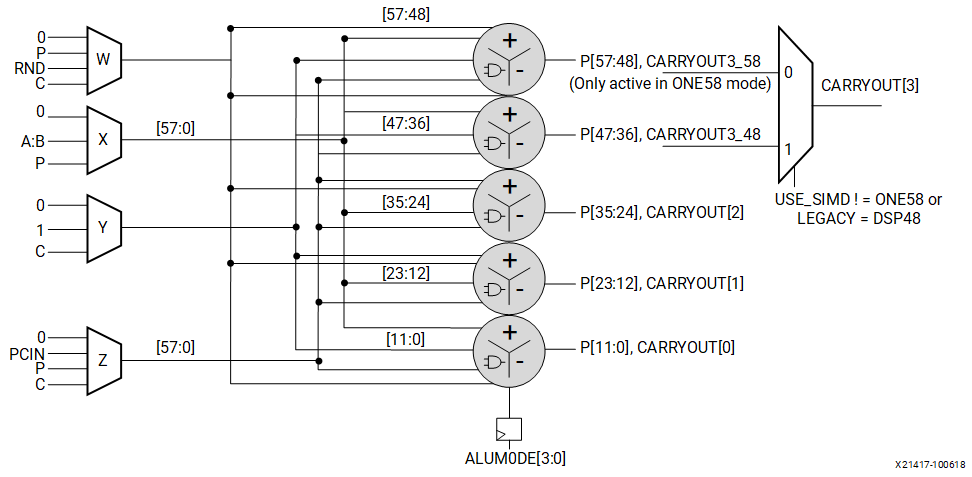# Single Instruction, Multiple Data (SIMD) Mode

## Versal ACAP DSP Engine Architecture Manual (AM004)

Document ID
AM004
Release Date
2022-09-11
Revision
1.2.1 English

The 58-bit adder/subtracter/accumulator can be split into smaller data segments where the internal carry propagation between segments is blocked to ensure independent operation for all segments. The adder/subtracter/accumulator can be split into four 12-bit adder/subtracter/accumulators or two 24-bit adder/subtracter/accumulators with carry out signal per segment. The SIMD mode segmentation is a static configuration as opposed to dynamic OPMODE type control (see the following figure). In all non-58-bit SIMD modes the upper 10-bits of the adder/subtracter/accumulator is disabled. This is done by driving the W/X/Y/Z inputs on the upper 10-bits to a static 0. This means that in TWO24 and FOUR12 modes the DSP58 operates the same as the DSP48E2, with the upper 10-bits of the P output equaling zero.

Figure 1. SIMD Adder Configuration• Four segments of dual, ternary, or quad adders with 12-bit inputs, a 12-bit output, and a carry output for each segment
• Function controlled dynamically by ALUMODE[3:0], and operand source by OPMODE[8:0]
• All four adder/subtracter/accumulators perform same function
• Two segments of dual, ternary, or quad adders with 24-bit inputs, a 24-bit output, and a carry output for each segment is also available (not pictured).

The SIMD feature allows the 58-bit logic unit to be split into multiple smaller logic units (see the figure above). Each smaller logic unit performs the same function. This function can also be changed dynamically through the ALUMODE[3:0] and opmode control inputs.

Note: The Carry-In input selected by the CARRYINSEL only propagates to the lowest-order adder in SIMD mode, that is, P[11:0] in FOUR12 and P[23:0] in TWO24 modes.